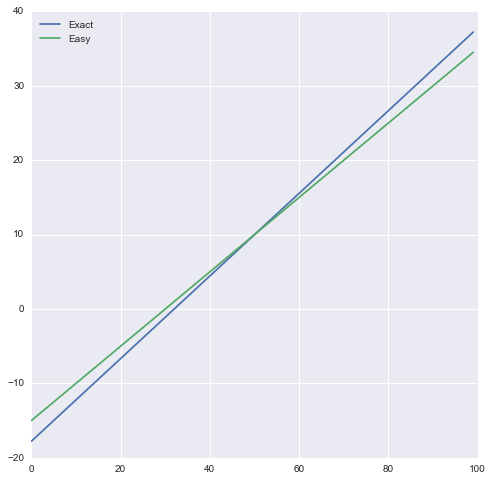# Sometimes less accurate models are better¶

Sometimes we don't always want the best model in terms of statistical fit, rather the best model for the circumstances in which we apply it. For example, often a less biased model is preferred over a best fit model if we plan to apply our model to many new samples.

I've been fortunate to have many European friends, and have picked up a few tricks from them, including an easy way to convert between Celsius and Farenheit. This is a good example of when a slightly less accurate model is more useful since it's easier to do the computations mentally.

First let's start off with the proper formulas, using the equation:

$$F = \frac{9}{5} C + 32$$
In :
def f_to_c(f_temp):
return (f_temp - 32) * 5. / 9

def c_to_f(c_temp):
return 9./5 * c_temp + 32


I can't speak for everyone, but multiplying by 5./9 in my head takes some effort, and it doesn't always work out nicely: $$\frac{5}{9} (80 - 32) = \frac{5}{9} * 48 = \frac{5}{9} * (5 * 9 + 3) = 25 + \frac{15}{9} = 26 + 6/9$$ which I would just round to 27.

In :
f_to_c(80)

Out:
26.666666666666668

Now let's look at the "easy formula":

$$F = 2 C + 30$$
In :
def easy_f_to_c(f_temp):
return (f_temp - 30) / 2.

def easy_c_to_f(c_temp):
return 2 * c_temp + 30

In :
easy_f_to_c(80)

Out:
25.0

Adding and substracting 30 and multiplying or diving by 2 is much easier mentally than trying to deal with $5/9$ or $9/5$. But how accurate is the result? In this case we found the temperature to be 25 C rather than 26.7 C, which seems ok for practical purposes, such as whether I'll need a jacket today.

Let's plot the functions over a typical range of tempatures from 0 to 100 degrees Farenheit.

In :
import matplotlib.pyplot as plt
import seaborn as sns

%matplotlib inline
# Make our figures larger
plt.rcParams["figure.figsize"] = (8, 8)

In :
temps = list(range(0, 100))
plt.plot(temps, map(f_to_c, temps), label="Exact")
plt.plot(temps, map(easy_f_to_c, temps), label="Easy")
plt.legend(loc=2)
plt.show()Looks pretty close! Let's fill out the following table, based on the common mnemonic for Celsius temperatures:

• 30 is hot
• 20 in nice
• 10 is cool
• 0 is ice
In :
import pandas as pd

data = [[30, "hot"], [20, "nice"], [10, "cool"], [0, "ice"]]
df = pd.DataFrame(data, columns=["Celsius", "Feeling"])

Out:
Celsius Feeling
0 30 hot
1 20 nice
2 10 cool
3 0 ice

Now let's try our two formulas to see if all the values match up to our expectations of how Farenheit temperatures feel.

In :
df["Farenheit"] = df["Celsius"].apply(c_to_f)
df["Easy Farenheit"] = df["Celsius"].apply(easy_c_to_f)

Not bad! And as a bonus we see that the two formulas yield the same value at 10 Celsius == 50 Farenheit. That means our easy conversions will be most accurate in the 30 to 70 Farenheit range, which we can see from the table. Moreover, I think most would agree that 86-90 F is a hot day well above room temperature of 72, close to nice.